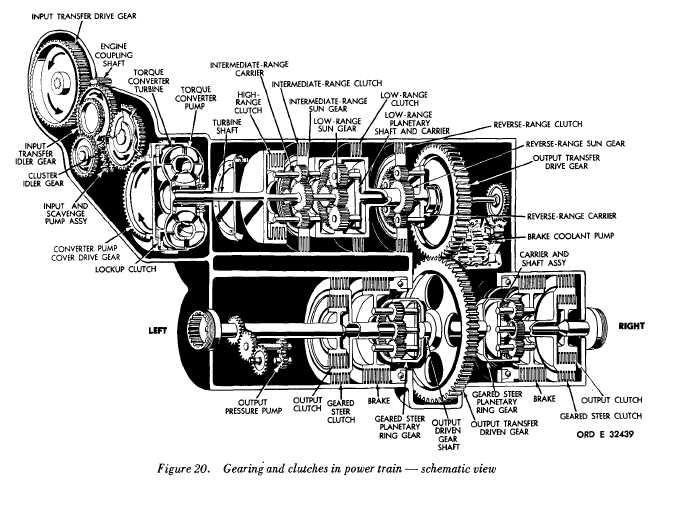FIGURE 20 GEARING AND CLUTCHES IN POWER TRAIN - SCHEMATIC VIEW
Custom SearchFigure 20 P A R   4 3 - 4 4 T O R Q U E     P A T H S C H A P   2,   SEC  V mitted   hydraulically   from   the   converter   pump t o     t h e     c o n v e r t e r     t u r b i n e .     W h e n     t h e     l o c k up clutch   is   engaged,   the   pump   and   turbine   are locked   together   and   torque   is   transmitted   me- c h a n i c a l l y . .   W h e n     t r a n s m i t t e d     h y d r a u l i c a l l y ,     t he torque   maybe   multiplied   as   much   as   3.3   times (at   turbine   stall).   When   transmitted   mechani- cally,   torque   leaving   the   converter   is   equal   to t h a t    e n t e r i n g    i t .    T h e    l o c k u p    c l u t c h    e n g a g es and    releases    automatically. .   At   lower   speeds,   when   high   torque   is r e q u i r e d ,     t h e     l o c k u p     c l u t c h     i s     r e l e a s e d .     At higher   speeds,   when   less   torque   is   required, the   lockup   clutch   is   engaged.   Lockup   engage- m e n t    c a n    o c c u r    i n    e v e r y    g e a r    r a n g e    a n d    in neutral.   In   figures   22   through   34   the   converter output   is   indicated   by   yellow   when   the   lockup clutch   is   released;   by   red   when   engaged. 4 4 .     T O R Q U E     P A T H     -     O U T P UT TRANSFER    GEARING    (fig.    20) .   Two   gears   makeup   the   output   transfer gearing.   The   drive   gear   is   splined   to   the   low- range   carrier   shaft   which   is   the   range   gearing output   for   the   power   train   regardless   of   which gear   is   engaged. The   drive   gear   meshes   with the   driven   gear   and   imparts   opposite   rotation. .   T h e     d r i v e n     g e a r     t r a n s m i t s     t o r q u e, through   a   splined   shaft,   to   the   steer   planetary ring   gears   on   either   side   of   the   driven   gear. The    speed    reduction,    and    corresponding    torque i n c r e a s e ,    i s    1 . 3 0    t o    1 . 0 0 . 4 7Integrated Publishing, Inc. - A (SDVOSB) Service Disabled Veteran Owned Small Business